Subsections

# NPT Ensemble

## The Problem

The constant volume ensemble is not very convenient for the study of phase transitions. Some experimental values, like the excess properties, are measured at constant pressure, not at constant volume, therefore it would be nice to have a method to simulate the isobaric conditions also. The average of the value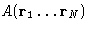in the NPT ensemble is given as: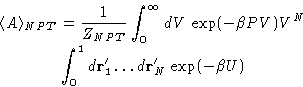here we again made the transformation to the primed'' coordinates, rescaling them by the box dimensions. Now we have to develop a Markov chain that samples the phase space with the probability distribution proportional to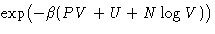.Metropolis algorithm is implemented in the same way as for NVT ensemble, but now we also allow the volume to change. We have two kinds of the Monte Carlo moves: particles displacement, performed the same way as in the NVT simulations, and Volume change. The acceptance criterium is, of course, modified now, as instead of the energy difference between the old and new states, it is based on the difference of enthalpies: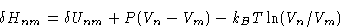(7)

## NPT MC algorithm

1.
Prepare the initial configuration of the system with the desired density (box size and number of particles). Usually just a simple cubic lattice works.
2.
Loop through the particles:
• Pick up a particle and decide its new position by choosing the displacement components at random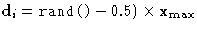(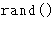is a random number from 0 to 1,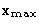is the maximum allowed displacement).
• Calculate the energy change, induced by the displacement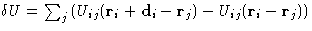and decide, whether accept it or not, according to the Metropolis criterium.
• If the new configuration is accepted, update position, energy, etc.
• Pick the next particle, etc.

3.
Make a volume change as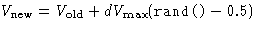. Calculate new energy of the system. and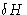from equation (7), and decide, whether to accept it or not, based on the Metropolis criterium.
4.
Calculate necessary averages, print out the results, etc. Go back to step 2.Next: Quiz Up: Calculation of Thermodynamic Properties. Previous: Thermodynamic Properties in NVT

© 1997 Boris Veytsman and Michael Kotelyanskii
Thu Nov 13 19:07:05 EST 1997